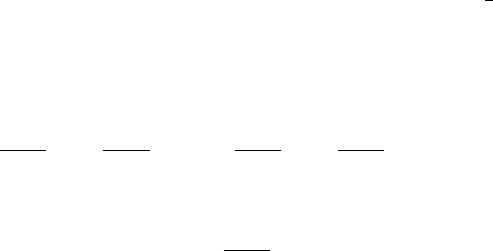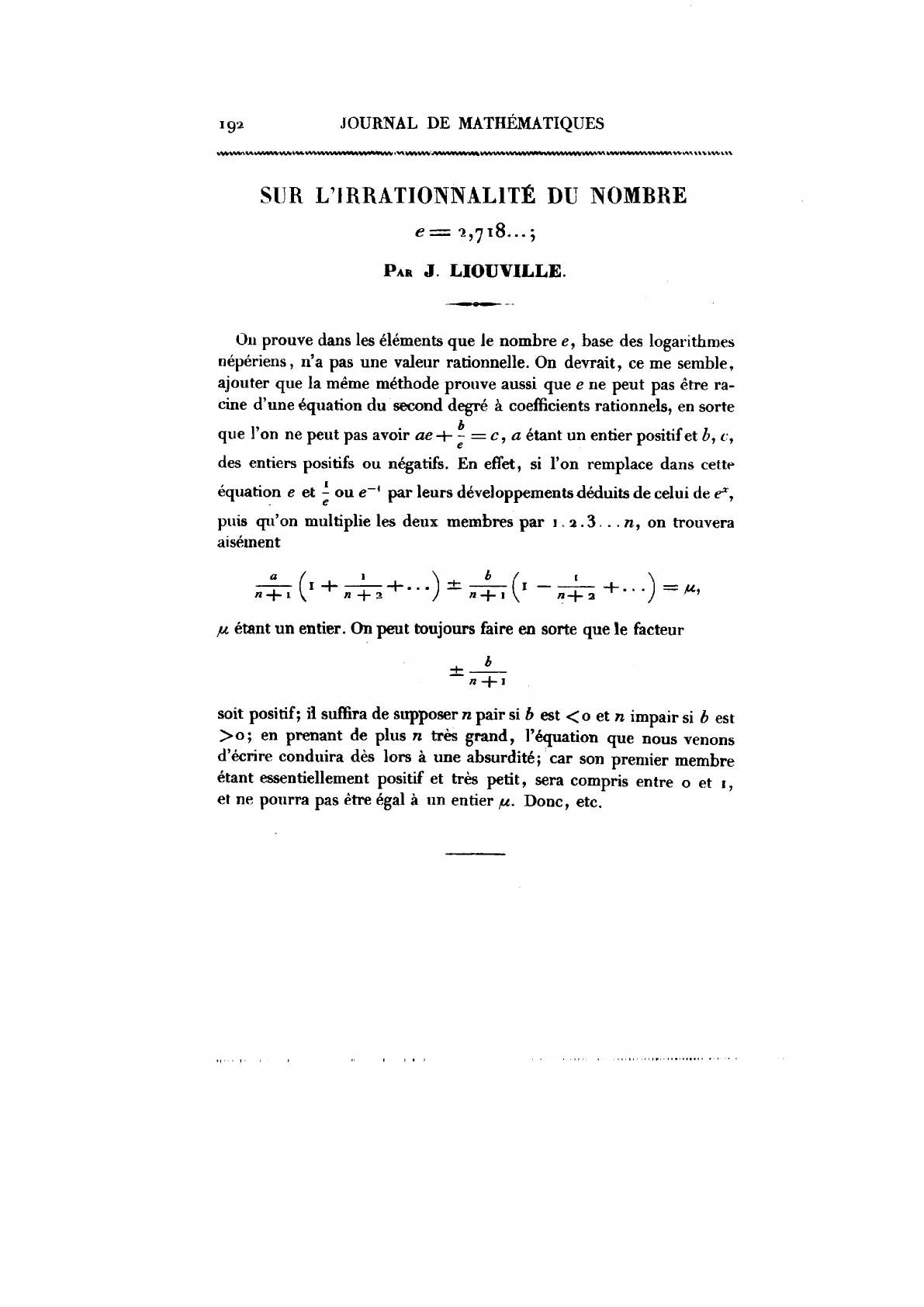Joseph Liouville (1809 – 1882) was one of the leading French mat...
Note that Liouville doesn't use the factorial symbol "!". Although ...
If we multiply both sides by $n!$ and we use $e^{x}= \sum_{l=0}^{\i... Liouville proves that$e$is not an algebraic number of degree 2 (i... This is the original paper published in the French journal "Journal...About the irrationality of e=2.718... J. Liouville 1840 We prove that e, basis of the neperien logarithm, is not a rational number. The same method can also be used to prove that e cannot be a root of a second degree equation with rational coecients, i.e. we cannot ﬁnd ae + b e = c,such that a is a positive integer and b, c are positive or negati ve integers. As a matter of fact, if we replace e and 1/e or e 1 with their power series deduced from e x , and given that we multiply both sides of the equation by 1 2 3 ... n,we ﬁnd that a n +1 1+ 1 n +2 + ... ± b n +1 1 1 n +2 + ... = µ where µ is an integer. We can make it so that ± b n +1 is positive; we just need to suppose that n is even when b<0 and t h at n is odd when b>0; for big values of n the equation above leads to a contradiction; the ﬁrst term of the equation is positive and very small, with values between 0 and 1, and as such can never be equal to an integer µ. So, etc. 1This is the original paper published in the French journal "Journal de Mathématiques" in 1840. Joseph Liouville (1809 – 1882) was one of the leading French mathematicians in the generation between Évariste Galois and Charles Hermite. In all, Liouville wrote over 400 mathematical papers, 200 in number theory alone. ![](https://www.ecured.cu/images/thumb/c/c8/JosephLiouville.png/260px-JosephLiouville.png) Liouville proves that$e$is not an algebraic number of degree 2 (in other words it's not a solution of 2nd degree polynomial with rational coefficients) but it's not difficult to see that if$c=0$we get for free the proof that$e^2$is irrational. $$e^2=\frac{b}{a} \equiv ae=be^{-1}$$ Note that Liouville doesn't use the factorial symbol "!". Although the notation n! was first introduced by Christian Kramp (1760-1826) in 1808 in his book "Éléments d'arithmétique universelle" it hadn't still been widely adopted by the mathematical community. If we multiply both sides by$n!$and we use$e^{x}= \sum_{l=0}^{\infty} \frac{x^k}{k!} $we get $$n!a\left(\sum_{k=0}^{n} \frac{1}{k!}+\sum_{k=n+1}^{\infty} \frac{1}{k!} \right) + n!b\left(\sum_{k=0}^{n} \frac{(-1)^{k}}{k!}+\sum_{k=n+1}^{\infty} \frac{(-1)^{k}}{k!} \right) = n!c \\ \sum_{k=n+1}^{\infty} \frac{n!a}{k!} + \sum_{k=n+1}^{\infty} \frac{(-1)^{k}n!b}{k!} = \underbrace{n!c + \sum_{k=0}^{n} \frac{n!a}{k!} + \sum_{k=0}^{n} \frac{(-1)^{k}n!b}{k!}}_{\mu}$$ Note that since$k<n$all the terms on the right hand side are integers and so$\mu$is also an integer. $$\sum_{k=n+1}^{\infty} \frac{n!a}{k!}=\frac{a}{n+1}\left(1+\frac{1}{n+2}+...\right) < a\left(\frac{1}{n+1}+\frac{1}{(n+1)^2}+...\right)=\frac{a}{n}$$ At the same time $$\sum_{k=n+1}^{\infty} \frac{(-1)^{k}n!b}{k!}=\frac{b(-1)^{n+1}}{n+1}\left(1- \frac{1}{n+2}+...\right)$$ Since $$\frac{1}{n+1}\left(1- \frac{1}{n+2}+...\right) < \frac{1}{n+1}\left(1+ \frac{1}{n+2}+...\right) < \frac{1}{n}$$ if we choose a combination of$b$and$n$so that$b(-1)^{n+1} > 0$we get $$0<\sum_{k=n+1}^{\infty} \frac{n!a}{k!} + \sum_{k=n+1}^{\infty} \frac{(-1)^{k}n!b}{k!}< \frac{a+b}{n}$$ In particular if we pick a large enough$n\$ we can get $$\frac{a+b}{n}<1$$ which generates a contradiction since on the left hand side of the equation we have something that is less than 1 and greater than 0 and on the right hand side we have an integer.